# forecast

Forecast conditional variances from conditional variance models

## Syntax

``V = forecast(Mdl,numperiods,Y0)``
``V = forecast(Mdl,numperiods,Y0,Name,Value)``

## Description

example

````V = forecast(Mdl,numperiods,Y0)` returns `numperiods` consecutive forecasted conditional variances `V` of the fully specified, univariate conditional variance model `Mdl`. The model `Mdl` can be a `garch`, `egarch`, or `gjr` model object. The presample response data `Y0` initializes the model to generate forecasts.```

example

````V = forecast(Mdl,numperiods,Y0,Name,Value)` generates forecasts with additional options specified by one or more name-value pair arguments. For example, you can initialize the model by specifying presample conditional variances.```

## Examples

collapse all

Forecast the conditional variance of simulated data over a 30-period horizon.

Simulate 100 observations from a GARCH(1,1) model with known parameters.

```Mdl = garch('Constant',0.02,'GARCH',0.8,'ARCH',0.1); rng default; % For reproducibility [v,y] = simulate(Mdl,100);```

Forecast the conditional variances over a 30-period horizon, with and without using the simulated data as presample innovations. Plot the forecasts.

```vF1 = forecast(Mdl,30,'Y0',y); vF2 = forecast(Mdl,30); figure plot(v,'Color',[.7,.7,.7]) hold on plot(101:130,vF1,'r','LineWidth',2); plot(101:130,vF2,':','LineWidth',2); title('Forecasted Conditional Variances') legend('Observed','Forecasts with Presamples',... 'Forecasts without Presamples','Location','NorthEast') hold off```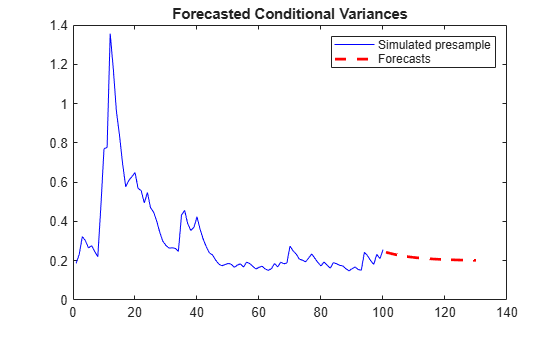Forecasts made without using presample innovations equal the unconditional innovation variance. Forecasts made using presample innovations converge asymptotically to the unconditional innovation variance.

Forecast the conditional variance of simulated data over a 30-period horizon.

Simulate 100 observations from an EGARCH(1,1) model with known parameters.

```Mdl = egarch('Constant',0.01,'GARCH',0.6,'ARCH',0.2,... 'Leverage',-0.2); rng default; % For reproducibility [v,y] = simulate(Mdl,100);```

Forecast the conditional variance over a 30-period horizon, with and without using the simulated data as presample innovations. Plot the forecasts.

```Vf1 = forecast(Mdl,30,y); Vf2 = forecast(Mdl,30); figure plot(v,'Color',[.7,.7,.7]) hold on plot(101:130,Vf1,'r','LineWidth',2); plot(101:130,Vf2,':','LineWidth',2); title('Forecasted Conditional Variances') legend('Observed','Forecasts with Presamples',... 'Forecasts without Presamples','Location','NorthEast') hold off```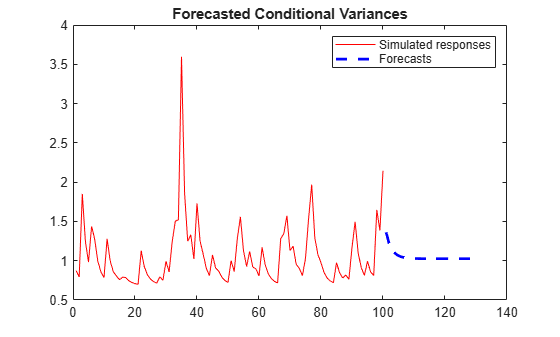Forecasts made without using presample innovations equal the unconditional innovation variance. Forecasts made using presample innovations converge asymptotically to the unconditional innovation variance.

Forecast the conditional variance of simulated data over a 30-period horizon.

Simulate 100 observations from a GJR(1,1) model with known parameters.

```Mdl = gjr('Constant',0.01,'GARCH',0.6,'ARCH',0.2,... 'Leverage',0.2); rng default; % For reproducibility [v,y] = simulate(Mdl,100);```

Forecast the conditional variances over a 30-period horizon, with and without using the simulated data as presample innovations. Plot the forecasts.

```vF1 = forecast(Mdl,30,'Y0',y); vF2 = forecast(Mdl,30); figure plot(v,'Color',[.7,.7,.7]) hold on plot(101:130,vF1,'r','LineWidth',2); plot(101:130,vF2,':','LineWidth',2); title('Forecasted Conditional Variances') legend('Observed','Forecasts with Presamples',... 'Forecasts without Presamples','Location','NorthEast') hold off```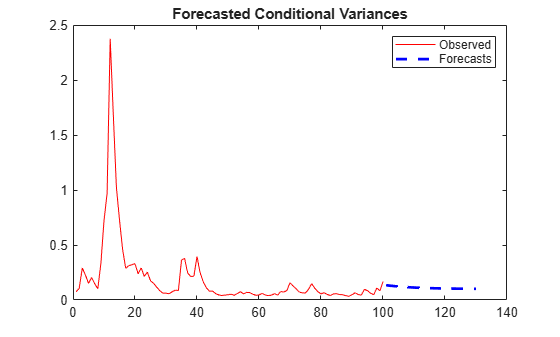Forecasts made without using presample innovations equal the unconditional innovation variance. Forecasts made using presample innovations converge asymptotically to the unconditional innovation variance.

Forecast the conditional variance of the NASDAQ Composite Index returns over a 500-day horizon using GARCH(1,1), EGARCH(1,1) and GJR(1,1) models.

Load the NASDAQ data included with the toolbox. Convert the index to returns. Plot the returns.

```load Data_EquityIdx nasdaq = DataTable.NASDAQ; r = price2ret(nasdaq); T = length(r); meanR = mean(r)```
```meanR = 4.7771e-04 ```
```figure; plot(dates(2:end),r,dates(2:end),meanR*ones(T,1),'--r'); datetick; title('Daily NASDAQ Returns'); xlabel('Day'); ylabel('Return');```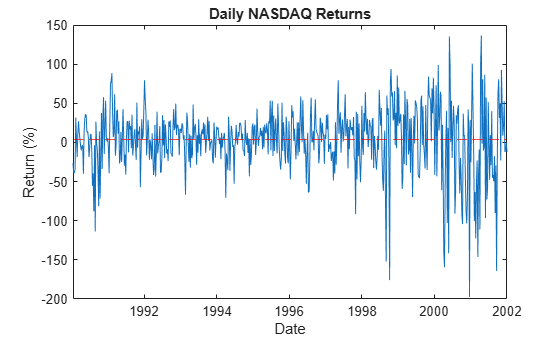The variance of the series seems to change. This change is an indication of volatility clustering. The conditional mean model offset is very close to zero.

Fit GARCH(1,1), EGARCH(1,1), and GJR(1,1) models to the data. By default, the software sets the conditional mean model offset to zero.

```MdlGARCH = garch(1,1); MdlEGARCH = egarch(1,1); MdlGJR = gjr(1,1); EstMdlGARCH = estimate(MdlGARCH,r); ```
``` GARCH(1,1) Conditional Variance Model (Gaussian Distribution): Value StandardError TStatistic PValue __________ _____________ __________ __________ Constant 2.0101e-06 5.4314e-07 3.7008 0.00021491 GARCH{1} 0.8833 0.0084528 104.5 0 ARCH{1} 0.10919 0.007662 14.251 4.4111e-46 ```
`EstMdlEGARCH = estimate(MdlEGARCH,r); `
``` EGARCH(1,1) Conditional Variance Model (Gaussian Distribution): Value StandardError TStatistic PValue _________ _____________ __________ __________ Constant -0.13494 0.022096 -6.1073 1.0135e-09 GARCH{1} 0.98389 0.0024225 406.15 0 ARCH{1} 0.19964 0.013964 14.297 2.2809e-46 Leverage{1} -0.060242 0.005646 -10.67 1.4067e-26 ```
`EstMdlGJR = estimate(MdlGJR,r);`
``` GJR(1,1) Conditional Variance Model (Gaussian Distribution): Value StandardError TStatistic PValue __________ _____________ __________ __________ Constant 2.4568e-06 5.6829e-07 4.3231 1.5383e-05 GARCH{1} 0.88144 0.0094781 92.997 0 ARCH{1} 0.06394 0.0091771 6.9674 3.2291e-12 Leverage{1} 0.088909 0.0099026 8.9784 2.7465e-19 ```

Forecast the conditional variance for 500 days using the fitted models. Use the observed returns as presample innovations for the forecasts.

```vFGARCH = forecast(EstMdlGARCH,500,r); vFEGARCH = forecast(EstMdlEGARCH,500,r); vFGJR= forecast(EstMdlGJR,500,r);```

Plot the forecasts along with the conditional variances inferred from the data.

```vGARCH = infer(EstMdlGARCH,r); vEGARCH = infer(EstMdlEGARCH,r); vGJR = infer(EstMdlGJR,r); datesFH = dates(end):(dates(end)+1000); % 1000 period forecast horizon figure; subplot(3,1,1); plot(dates(end-250:end),vGARCH(end-250:end),'b',... datesFH(2:end-500),vFGARCH,'b--'); legend('Inferred','Forecast','Location','NorthEast'); title('GARCH(1,1) Conditional Variances'); datetick; axis tight; subplot(3,1,2); plot(dates(end-250:end),vEGARCH(end-250:end),'r',... datesFH(2:end-500),vFEGARCH,'r--'); legend('Inferred','Forecast','Location','NorthEast'); title('EGARCH(1,1) Conditional Variances'); datetick; axis tight; subplot(3,1,3); plot(dates(end-250:end),vGJR(end-250:end),'k',... datesFH(2:end-500),vFGJR,'k--'); legend('Inferred','Forecast','Location','NorthEast'); title('GJR(1,1) Conditional Variances'); datetick; axis tight;```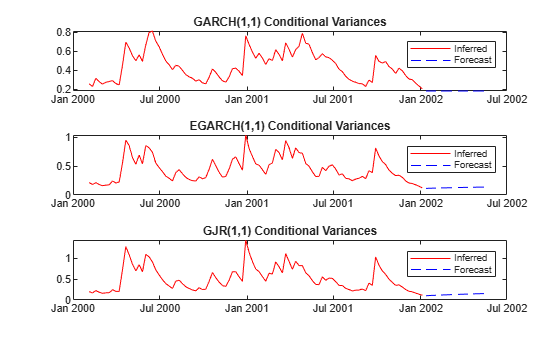Plot conditional variance forecasts for 1000 days.

```vF1000GARCH = forecast(EstMdlGARCH,1000,r); vF1000EGARCH = forecast(EstMdlEGARCH,1000,r); vF1000GJR = forecast(EstMdlGJR,1000,r); figure; plot(datesFH(2:end),vF1000GARCH,'b',... datesFH(2:end),vF1000EGARCH,'r',... datesFH(2:end),vF1000GJR,'k'); legend('GARCH','EGARCH','GJR','Location','NorthEast'); title('Conditional Variance Forecast Asymptote') datetick;```The forecasts converge asymptotically to the unconditional variances of their respective processes.

## Input Arguments

collapse all

Conditional variance model without any unknown parameters, specified as a `garch`, `egarch`, or `gjr` model object.

`Mdl` cannot contain any properties that have `NaN` value.

Forecast horizon, or the number of time points in the forecast period, specified as a positive integer.

Data Types: `double`

Presample response data used to infer presample innovations `E0`, and whose conditional variance is forecasted, specified as a numeric column vector with length `numpreobs` or a `numpreobs`-by-`numpaths` numeric matrix.

`Y0` can represent a mean 0 presample innovations series with a variance process characterized by the conditional variance model `Mdl`. `Y0` can also represent a presample innovations series plus an offset (stored in `Mdl.Offset`). For more details, see Algorithms.

Rows of `Y0` correspond to periods in the presample, and the last row contains the latest presample response. `numpreobs` is the number of specified presample responses and it must be at least `Mdl.Q` to initialize the variance equation. If `numpreobs` exceeds `Mdl.Q`, `forecast` uses only the latest `Mdl.Q` rows. For more details, see Time Base Partitions for Forecasting.

Columns of `Y0` correspond to separate, independent paths.

• If `Y0` is a column vector, `forecast` applies it to each forecasted path. In this case, all forecast paths `Y` derive from the same initial responses.

• If `Y0` is a matrix, it must have `numpaths` columns, where `numpaths` is the maximum among the second dimensions of the specified presample observation arrays `Y0` and `V0`.

Data Types: `double`

### Name-Value Arguments

Specify optional comma-separated pairs of `Name,Value` arguments. `Name` is the argument name and `Value` is the corresponding value. `Name` must appear inside quotes. You can specify several name and value pair arguments in any order as `Name1,Value1,...,NameN,ValueN`.

Example: `'V0',[1 0.5;1 0.5]` specifies two different presample paths of conditional variances.

Presample conditional variances used to initialize the conditional variance model, specified as the comma-separated pair consisting of `'V0'` and a numeric column vector or matrix with positive entries and `numpaths` columns.

Rows of `V0` correspond to periods in the presample, and the last row contains the latest presample conditional variance.

• For GARCH(P,Q) and GJR(P,Q) models, `V0` must have at least `Mdl.P` rows to initialize the variance equation.

• For EGARCH(P,Q) models, `V0` must have at least `max([Mdl.P Mdl.Q])` rows to initialize the variance equation.

If the number of rows exceeds the minimum number, then `forecast` uses the latest observations only.

Columns of `V0` correspond to separate, independent paths.

• If `V0` is a column vector, `forecast` applies it to each forecasted path. In this case, the conditional variance model of all forecast paths `Y` derives from the same initial conditional variances.

• If `V0` is a matrix, it must have `numpaths` columns.

• By default, if `Y0` has at least max(P,Q) + P rows, `forecast` infers any necessary presample conditional variances by passing `Mdl` and `Y0` to `infer`.

• By default, if `Y0` has insufficient length:

• For GARCH(P,Q) and GJR(P,Q) models, `forecast` sets any necessary presample conditional variances to the unconditional variance of the conditional variance process.

• For EGARCH(P,Q) models, `forecast` sets any necessary presample conditional variances to the exponentiated, unconditional mean of the logarithm of the EGARCH(P,Q) variance process.

Data Types: `double`

Note

`forecast` assumes that you synchronize presample data such that the latest observation of each presample series occurs simultaneously.

## Output Arguments

collapse all

Minimum mean square error (MMSE) forecasts of conditional variances of future model innovations, returned as a numeric column vector with length `numperiods` or a `numperiods`-by-`numpaths` numeric matrix. `V` represents a continuation of `V0` (`V(1,:)` occurs in the next time point after `V0(end,:)`).

`V(j,k)` contains the `j`-period-ahead forecasted conditional variance of path `k`.

`forecast` determines `numpaths` from the number of columns in the presample data sets `Y0` and `V0`. For details, see Algorithms. If each presample data set has one column, then `V` is a column vector.

collapse all

### Time Base Partitions for Forecasting

Time base partitions for forecasting are two disjoint, contiguous intervals of the time base; each interval contains time series data for forecasting a dynamic model. The forecast period (forecast horizon) is a `numperiods` length partition at the end of the time base during which `forecast` generates forecasts `V` from the dynamic model `Mdl`. The presample period is the entire partition occurring before the forecast period. `forecast` can require observed responses (or innovations) `Y0` or conditional variances `V0` in the presample period to initialize the dynamic model for forecasting. The model structure determines the types and amounts of required presample observations.

A common practice is to fit a dynamic model to a portion of the data set, then validate the predictability of the model by comparing its forecasts to observed responses. During forecasting, the presample period contains the data to which the model is fit, and the forecast period contains the holdout sample for validation. Suppose that yt is an observed response series. Consider forecasting conditional variances from a dynamic model of yt `numperiods` = K periods. Suppose that the dynamic model is fit to the data in the interval [1,TK] (for more details, see `estimate`). This figure shows the time base partitions for forecasting.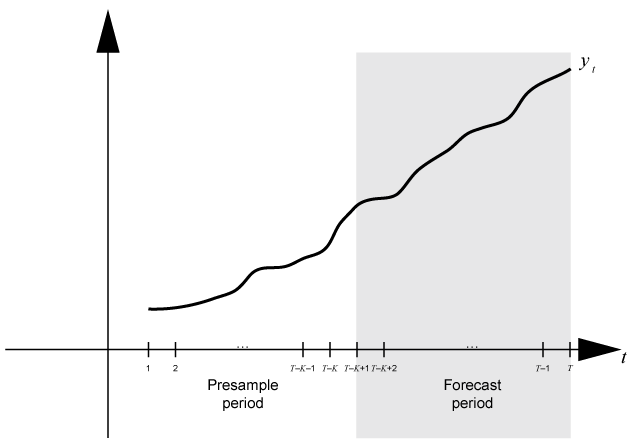For example, to generate forecasts `Y` from a GARCH(0,2) model, `forecast` requires presample responses (innovations) `Y0` = ${\left[\begin{array}{cc}{y}_{T-K-1}& {y}_{T-K}\end{array}\right]}^{\prime }$ to initialize the model. The 1-period-ahead forecast requires both observations, whereas the 2-periods-ahead forecast requires yTK and the 1-period-ahead forecast `V(1)`. `forecast` generates all other forecasts by substituting previous forecasts for lagged responses in the model.

Dynamic models containing a GARCH component can require presample conditional variances. Given enough presample responses, `forecast` infers the required presample conditional variances. This figure shows the arrays of required observations for this case, with corresponding input and output arguments.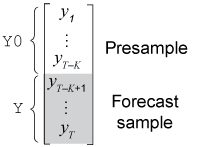## Algorithms

• If the conditional variance model `Mdl` has an offset (`Mdl.Offset`), `forecast` subtracts it from the specified presample responses `Y0` to obtain presample innovations `E0`. Subsequently, `forecast` uses `E0` to initialize the conditional variance model for forecasting.

• `forecast` sets the number of sample paths to forecast `numpaths` to the maximum number of columns among the presample data sets `Y0` and `V0`. All presample data sets must have either `numpaths` > 1 columns or one column. Otherwise, `forecast` issues an error. For example, if `Y0` has five columns, representing five paths, then `V0` can either have five columns or one column. If `V0` has one column, then `forecast` applies `V0` to each path.

• `NaN` values in presample data sets indicate missing data. `forecast` removes missing data from the presample data sets following this procedure:

1. `forecast` horizontally concatenates the specified presample data sets `Y0` and `V0` such that the latest observations occur simultaneously. The result can be a jagged array because the presample data sets can have a different number of rows. In this case, `forecast` prepads variables with an appropriate amount of zeros to form a matrix.

2. `forecast` applies list-wise deletion to the combined presample matrix by removing all rows containing at least one `NaN`.

3. `forecast` extracts the processed presample data sets from the result of step 2, and removes all prepadded zeros.

List-wise deletion reduces the sample size and can create irregular time series.

## Compatibility Considerations

expand all

 Bollerslev, T. “Generalized Autoregressive Conditional Heteroskedasticity.” Journal of Econometrics. Vol. 31, 1986, pp. 307–327.

 Bollerslev, T. “A Conditionally Heteroskedastic Time Series Model for Speculative Prices and Rates of Return.” The Review of Economics and Statistics. Vol. 69, 1987, pp. 542–547.

 Box, G. E. P., G. M. Jenkins, and G. C. Reinsel. Time Series Analysis: Forecasting and Control. 3rd ed. Englewood Cliffs, NJ: Prentice Hall, 1994.

 Enders, W. Applied Econometric Time Series. Hoboken, NJ: John Wiley & Sons, 1995.

 Engle, R. F. “Autoregressive Conditional Heteroskedasticity with Estimates of the Variance of United Kingdom Inflation.” Econometrica. Vol. 50, 1982, pp. 987–1007.

 Glosten, L. R., R. Jagannathan, and D. E. Runkle. “On the Relation between the Expected Value and the Volatility of the Nominal Excess Return on Stocks.” The Journal of Finance. Vol. 48, No. 5, 1993, pp. 1779–1801.

 Hamilton, J. D. Time Series Analysis. Princeton, NJ: Princeton University Press, 1994.

 Nelson, D. B. “Conditional Heteroskedasticity in Asset Returns: A New Approach.” Econometrica. Vol. 59, 1991, pp. 347–370.